Line: 1 to 1

# TWiki Site-Level Preferences

This topic defines site-level settings that apply to all users and webs on this TWikiSite.

Line: 49 to 49

• Note: Use the `[[...][...]]` syntax to link topics in case you disabled WikiWord linking. The `<noautolink> ... </noautolink>` syntax can be used to prevents links within a block of text.
>
>
• Use topic title instead of topic name in forced links, such as in link `[[BugItem1234]]`. If enabled, the link label is set to the form field named "Title", the topic preferences setting named TITLE, or the topic name if neither exists. Forced links with label such as `[[BugItem1234][bug 1234]]` are not affected. The topic title can be shown even with disabled flag by using syntax `[[+BugItem1234]]`. NOTE: Page rendering may be slower if enabled and if there are many forced links in a topic.

• If you use non WikiWords for topic names (for example "Expenses" or "Mighty1") and if you use bracket notation to link to those topics, it is useful to turn of all warnings (like: "WARNING: This topic name is not recommended because it will not be linked automatically") and checkboxes ("Allow non WikiWord for the new topic name"). Can be overwritten by web preferences. Set to `on` to activate:
• Set HIDE_NON_WIKI_WORD_WARNINGS =

Line: 71 to 74

• If not set: nothing
• Possible values: any string, for example `On this page:`
• Can be overridden by `title` parameter of variable TOC
>
>
• The default TOC style:
• Set TOC_STYLE =
• If not set: nothing
• Possible values: any CSS directive, for example `float: right; border: dotted gray 1px;`
• Can be overridden by `style` parameter of variable TOC

• Do not show a TOC if the topic it contains is included in another topic:
• Set TOC_HIDE_IF_INCLUDED =
• If not set: included TOC is shown
Line: 92 to 100

>
>
• In the user profile page, show the tag cloud instead of the watchlist changes in case the WatchlistPlugin is installed and enabled.
• #Local TAGCLOUDPROFILE = on

• Horizontal size of text edit box:
• Set EDITBOXWIDTH = 70
Line: 139 to 150

Changed:
<
<
•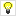NOTE: To automatically list all non-hidden webs in alphabetical order, use the `%WEBLIST{}%` variable instead:
Forum | Main | NDSG | ORUA | OpsRes | Sandbox | TWiki
>
>
•NOTE: To automatically list all non-hidden webs in alphabetical order, use the `%WEBLIST{}%` variable instead:
Forum | Main | NDSG | ORUA | OpsRes | Sandbox | TWiki

• Maximum size of FileAttachments in KB, 0 for no limit:
• Set ATTACHFILESIZELIMIT = 10000
Line: 162 to 173

• Set SEARCHVARDEFAULTTYPE = literal
• Stop words, e.g., common words and characters to exclude from a keyword search:
• Set SEARCHSTOPWORDS = a, all, am, an, and, any, as, at, b, be, by, c, d, e, for, from, g, h, how, i, i'm, i.e., in, is, isn't, it, it's, its, j, k, l, m, n, o, of, on, or, p, q, r, s, t, that, that's, the, this, to, u, v, w, was, wasn't, what, what's, when, where, who, who's, will, with, x, y, z
>
>

### E-mail Settings

Line: 240 to 253

• Legacy settings:
• Set INSTALLEDPLUGINS = The INSTALLEDPLUGINS variable is no longer supported. You can set the Plugins execution order using the configure interface.
>
>

>
>

## Parameterized Variables

ParameterizedVariables for global use are defined here.

• TWikiDashboardAddOn variable, documented in VarDASHBOARD:
• Set DASHBOARD = %INCLUDE{ "TWiki.TWikiDashboardAddOn" section="%section%" %IF{ "'%height{ default="" }%'!=''" then="height=\"%height%\"" }% %IF{ "'%width{ default="" }%'!=''" then="width=\"%width%\"" }% %IF{ "'%ENCODE{ "%image{ default="" }%" type="entity" }%'!=''" then="image=\"%image%\"" }% %IF{ "'%ENCODE{ "%title{ default="" }%" type="entity" }%'!=''" then="title=\"%title%\"" }% %IF{ "'%ENCODE{ "%button1{ default="" }%" type="entity" }%'!=''" then="button1=\"%button1%\"" }% %IF{ "'%ENCODE{ "%button2{ default="" }%" type="entity" }%'!=''" then="button2=\"%button2%\"" }% %IF{ "'%ENCODE{ "%button3{ default="" }%" type="entity" }%'!=''" then="button3=\"%button3%\"" }% %IF{ "'%ENCODE{ "%button4{ default="" }%" type="entity" }%'!=''" then="button4=\"%button4%\"" }% %IF{ "'%ENCODE{ "%button5{ default="" }%" type="entity" }%'!=''" then="button5=\"%button5%\"" }% %IF{ "'%ENCODE{ "%button6{ default="" }%" type="entity" }%'!=''" then="button6=\"%button6%\"" }% %IF{ "'%ENCODE{ "%button7{ default="" }%" type="entity" }%'!=''" then="button7=\"%button7%\"" }% %IF{ "'%ENCODE{ "%button8{ default="" }%" type="entity" }%'!=''" then="button8=\"%button8%\"" }% %IF{ "'%ENCODE{ "%style{ default="" }%" type="entity" }%'!=''" then="style=\"%style%\"" }% %IF{ "'%ENCODE{ "%titlestyle{ default="" }%" type="entity" }%'!=''" then="titlestyle=\"%titlestyle%\"" }% %IF{ "'%ENCODE{ "%contentstyle{ default="" }%" type="entity" }%'!=''" then="contentstyle=\"%contentstyle%\"" }% }%

• UserReports variable, documented in VarUSERREPORT:
• Set USERREPORT = %INCLUDE{ "TWiki.UserReports" section="%action%" %IF{ "'%ENCODE{ "%search{ default="~~" }%" type="entity" }%'!='~~'" then="search=\"%search%\"" }% %IF{ "'%limit{ default="~~" }%'!='~~'" then="limit=\"%limit%\"" }% %IF{ "'%order{ default="~~" }%'!='~~'" then="order=\"%order%\"" }% %IF{ "'%sort{ default="~~" }%'!='~~'" then="sort=\"%sort%\"" }% %IF{ "'%reverse{ default="~~" }%'!='~~'" then="reverse=\"%reverse%\"" }% %IF{ "'%user{ default="~~" }%'!='~~'" then="user=\"%user%\"" }% %IF{ "'%users{ default="~~" }%'!='~~'" then="users=\"%users%\"" }% %IF{ "'%selected{ default="~~" }%'!='~~'" then="selected=\"%selected%\"" }% %IF{ "'%height{ default="~~" }%'!='~~'" then="height=\"%height%\"" }% %IF{ "'%width{ default="~~" }%'!='~~'" then="width=\"%width%\"" }% %IF{ "'%ENCODE{ "%title{ default="~~" }%" type="entity" }%'!='~~'" then="title=\"%title%\"" }% %IF{ "'%name{ default="~~" }%'!='~~'" then="name=\"%name%\"" }% %IF{ "'%columns{ default="~~" }%'!='~~'" then="columns=\"%columns%\"" }% %IF{ "'%ENCODE{ "%style{ default="~~" }%" type="entity" }%'!='~~'" then="style=\"%style%\"" }% %IF{ "'%ENCODE{ "%firstoption{ default="~~" }%" type="entity" }%'!='~~'" then="firstoption=\"%firstoption%\"" }% %IF{ "'%ENCODE{ "%firstvalue{ default="~~" }%" type="entity" }%'!='~~'" then="firstvalue=\"%firstvalue%\"" }% }%
• Set USERSIG =
• Set BUBBLESIG =

## Rendering Shortcuts Settings

Changed:
<
<
• Line break and bullet with different levels of indentation:
>
>
These TWikiRenderingShortcuts facilitate doc writing.

• Set BB =
• Set BB2 =
•
• Set BB3 =
•
• Set BB4 =
•
Changed:
<
<
• Line break: Note that the setting has an intentional trailing space
>
>
• Line break: Note that the setting has an intentional trailing space

• Set BR =
Changed:
<
<
• Bullet sign:
>
>

• Set BULLET = •
Changed:
<
<
• Vertical caret symbol:
>
>

• Set CARET = ^
Changed:
<
<
• URL of current topic
>
>

• Add `%PARENTBC%` to a heading of a topic to show the breadcrumb of its parents. Parent topics are linked, topic names are shown spaced out in a smaller font, and are separated by `»` quotes. In addition, a `parentlist` SetGetPlugin variable is set to the parent list, and a `parent` variable is set to the immediate parent. This can be retrieved later in the topic using `%GET{parentlist}%` and `%GET{parent}%`, respectively.
• Example raw text:
`---+ %PARENTBC% Myself and me`
• Expands to:

# Grandma And Granpa » Mother And Father » Myself and me

• Set PARENTBC = »

Changed:
<
<
• Vertical bar / pipe symbol:
>
>

• Set VBAR = |

• Hint: Above shortcuts are useful in TWiki tables where you cannot use newlines
Line: 284 to 328

• Set S =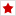• Set T =• Set U =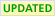>
>
• Set WIP =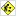• Set X =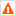• Set Y =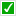Line: 387 to 432

• Format of a NewWikiWord link:
• Note that `\$text` is the topic link text, `\$summary` is the HTML-cleaned topic link text, `\$topic` is the name of the new topic, and `\$web` is the name of the web for the new topic. Values are taken from the link syntax `[[\$web.\$topic][\$text]]`. For normal plain WikiWord links `\$text` and `\$topic` are the same. The value of `\$web` is the current web unless the link is in the format `\$web.\$topic`.
• default setting:
Changed:
<
<
>
>

• alternative setting:
Changed:
<
<
>
>

• User signature format. The SIGNATUREFORMAT is used when creating new topics, BUBBLESIGNATUREFORMAT is used when adding comments with CommentPlugin:
• default setting:
• Set BUBBLESIGNATUREFORMAT = --
• Set SIGNATUREFORMAT = --
• Note: The USERSIG is defined in section "user_sig" of UserReports. The desired expanded signature format in a topic is `%USERSIG{%WIKINAME% - %DATE%}%`, this with unexpanded USERSIG, but expanded WIKINAME and DATE. A hack using CALCULATE is used to keep USERSIG unexpanded: `%CALCULATE{%U}%SERSIG{...}%`
• alternative legacy setting:
• #Set SIGNATUREFORMAT = -- TWikiGuest - 2023-06-04

• PreviewBackground image:
• Set PREVIEWBGIMAGE = /pub/TWiki/PreviewBackground/preview2bg.gif
Line: 426 to 479

## Protected System Settings

Changed:
<
<
The following settings are declared final to protect them as read only. These are system variables that should never be attempted set or altered in any preference topic or topic settings.
>
>
The following settings are declared final to protect them as read only. These are system variables that should never be attempted set or altered in any preference topic or topic settings.

• Set FINALPREFERENCES = WEB, TOPIC